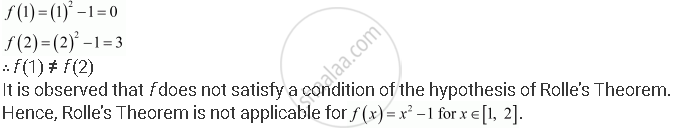Share

# Examine If Rolle’S Theorem is Applicable to Any of the Following Functions. Can You Say Some Thing About the Converse of Rolle’S Theorem from These Examples? F (X) = X2 – 1 for X ∈ [1, 2] - CBSE (Commerce) Class 12 - Mathematics

#### Question

Examine if Rolle’s Theorem is applicable to any of the following functions. Can you say some thing about the converse of Rolle’s Theorem from these examples?

f (x) = x2 – 1 for x ∈ [1, 2]

#### Solution

By Rolle’s Theorem, for a function f: [a, b] → R, if

(a) f is continuous on [a, b]

(b) f is differentiable on (a, b)

(c) f (a) = f (b)

then, there exists some c ∈ (a, b) such that f'(c) = 0

Therefore, Rolle’s Theorem is not applicable to those functions that do not satisfy any of the three conditions of the hypothesis.

f (x) = x2 – 1 for x ∈ [1, 2]

It is evident that f, being a polynomial function, is continuous in [1, 2] and is differentiable in (1, 2).Is there an error in this question or solution?

#### APPEARS IN

NCERT Solution for Mathematics Textbook for Class 12 (2018 to Current)
Chapter 5: Continuity and Differentiability
Q: 2.3 | Page no. 186

#### Video TutorialsVIEW ALL 

Solution Examine If Rolle’S Theorem is Applicable to Any of the Following Functions. Can You Say Some Thing About the Converse of Rolle’S Theorem from These Examples? F (X) = X2 – 1 for X ∈ [1, 2] Concept: Mean Value Theorem.
S